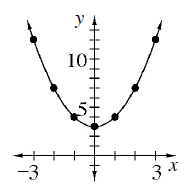### Home > CCA > Chapter 1 > Lesson 1.1.3 > Problem1-25

1-25.

Freda Function has another quadratic function for you to investigate! Graph the equation $y=x^2+3$ and then answer the questions from problem 1‑23.

Look at the equation. Which way do you think the parabola will open up?Below are the questions from problem 1-23 for you to answer.
• Are there $x$- and $y$-intercepts?  What are they?  Are there any intercepts that you expected but do not exist for your parabola?